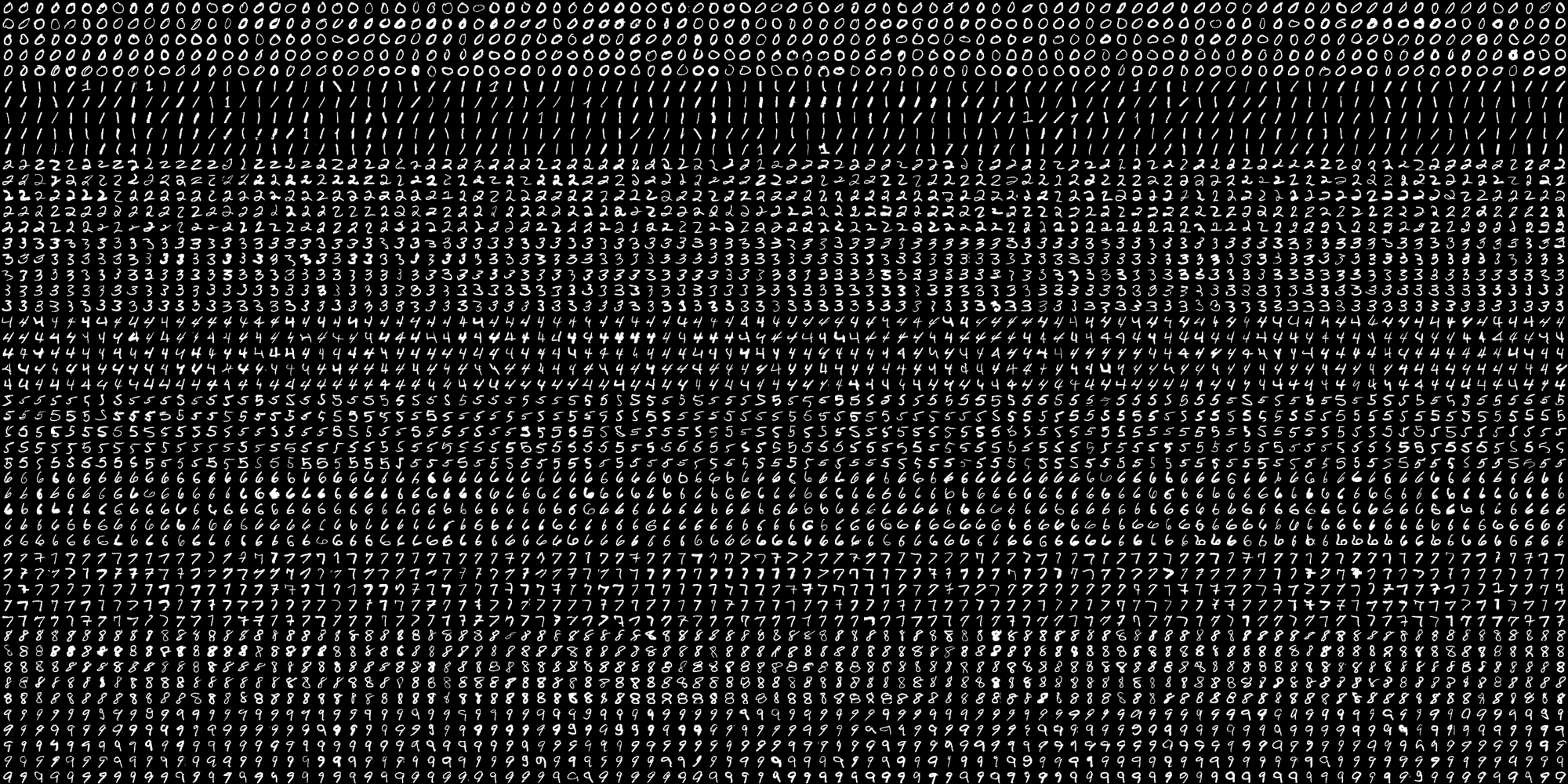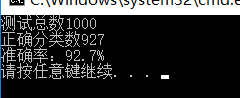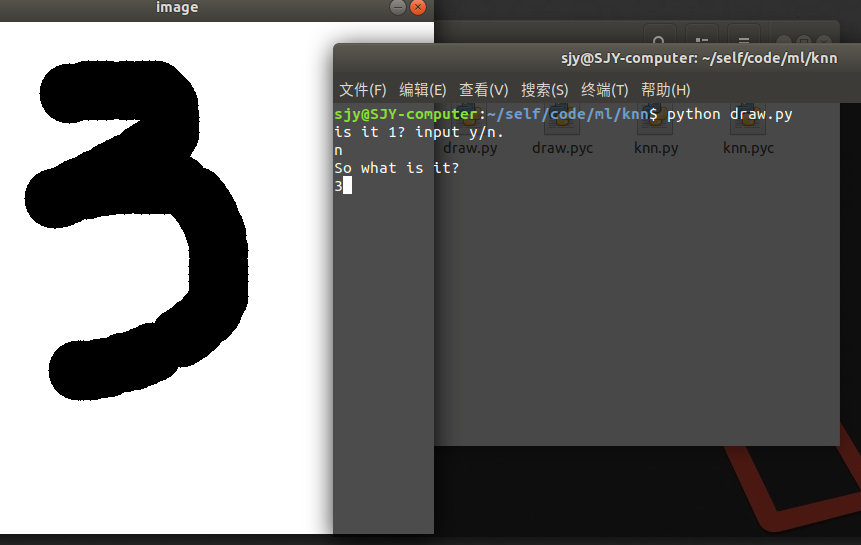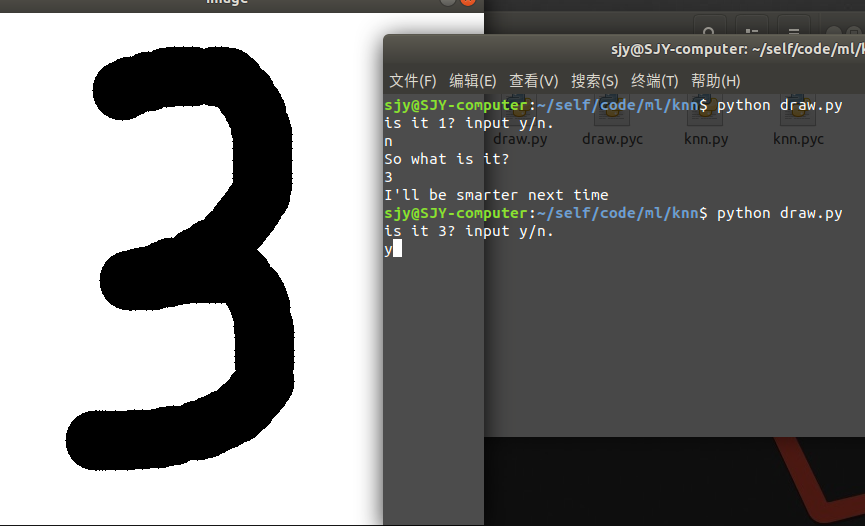• 机器学习入门KNN算法实现的手写数字识别 基本上照搬了http://lib.csdn.net/article/opencv/30167的代码，只是改了一点bug和增加了一点功能 输入就是直接在一个512*512大小的白色画布上画黑线，然后转化为01矩阵，用...
• 电脑通过串口传给单片机，单片机用KNN算法给出手写数字的预测结果，并且在液晶上显示
• 自己做课程设计时写的matlab程序，可运行，无error。有代码注释，易上手。相比网上的其它版本，我写的这个程序比较简单，适合新手，阅读门槛低，而且最重要的是可运行！大话不敢多说，绝对是网上最亲民的版本！...
• KNN算法使用MNIST数据集、0-1二值图集、自写的数字图片集，做手写数字识别的代码，文件夹分类明确。
• 图片大小为1000*2000,有0-9的10个数字，每5行为一个数字，总共50行，共有5000个手写数字，每个数字块大小为20*20。 为了后续方便处理，我们先写一段小程序把这5000个图截取出来：#include #incl
学习KNN（一） 图像分类与KNN原理 学习KNN（二）KNN算法手写数字识别的OpenCV实现 学习KNN（三）KNN+HOG实现手写数字识别
在OpenCV的安装文件路径/opencv/sources/samples/data/digits.png下，有这样一张图：图片大小为10002000,有0-9的10个数字，每5行为一个数字，总共50行，共有5000个手写数字，每个数字块大小为2020。 为了后续方便处理，我们先写一段小程序把这5000个图截取出来：
#include <opencv2/opencv.hpp>
#include <iostream>

using namespace std;
using namespace cv;

int main()
{
int  filename = 0,filenum=0;
Mat gray;
cvtColor(img, gray, CV_BGR2GRAY);
int b = 20;
int m = gray.rows / b;   //原图为1000*2000
int n = gray.cols / b;   //裁剪为5000个20*20的小图块

for (int i = 0; i < m; i++)
{
int offsetRow = i*b;  //行上的偏移量
if(i%5==0&&i!=0)
{
filename++;
filenum=0;
}
for (int j = 0; j < n; j++)
{
int offsetCol = j*b; //列上的偏移量
//截取20*20的小块
Mat tmp;
gray(Range(offsetRow, offsetRow + b), Range(offsetCol, offsetCol + b)).copyTo(tmp);
}
}
return 0;
}

截取之后每个以数字命名的文件夹内就都有500张图片了，需要注意的是OpenCV的imwrite是没有自动创建文件夹功能的，所以路径应该提前存在。
OpenCV提供的KNN算法构造函数：
C++: CvKNearest::CvKNearest()
C++: CvKNearest::CvKNearest(const Mat& trainData, const Mat& responses, const Mat& sam-
pleIdx=Mat(), bool isRegression=false, int max_k=32 )

训练函数为：
C++: bool CvKNearest::train(
const Mat& trainData, //训练数据
const Mat& responses,//对应的响应值
const Mat& sampleIdx=Mat(),//样本索引
bool isRegression=false,//是否是回归，否则是分类问题
int maxK=32, //最大K值
bool updateBase=false//是否更新数据，是，则maxK需要小于原数据大小 )

查找函数为：
C++: float CvKNearest::find_nearest(
const Mat& samples,//按行存储的测试数据
int k, //K 值
Mat* results=0,//预测结果
const float** neighbors=0, //近邻指针向量
Mat* neighborResponses=0, //近邻值
Mat* dist=0 //距离矩阵) const

C++: float CvKNearest::find_nearest(
const Mat& samples,
int k,
Mat& results,
Mat& neighborResponses,
Mat& dists) const

但是由于KNN的特点，其实并没有train的功能，所以train只是相当于存储下来训练样本，而且会在执行构造函数中执行训练过程。
在之前，我们已经把5000张图分别放进了10个文件夹里了，现在我们把其中的每个类别中前400张拿出来做训练数据，其余的测试，代码如下：
#include <iostream>
#include <opencv2/opencv.hpp>
#include <opencv2/ml/ml.hpp>
using namespace std;
using namespace cv;

int main()
{
Mat traindata ,trainlabel;
int k=5,testnum=0,truenum=0;
//读取训练数据 4000张
for (int i = 0; i < 10; i++)
{
for (int j =0;j<400;j++)
{
srcimage = srcimage.reshape(1,1);
traindata.push_back(srcimage);
trainlabel.push_back(i);
}
}
traindata.convertTo(traindata,CV_32F);
CvKNearest knn( traindata, trainlabel, cv::Mat(), false, k );
cv::Mat nearests( 1, k, CV_32F);
//读取测试数据  1000张
for (int i = 0; i < 10; i++)
{
for (int j =400;j<500;j++)
{
testnum++;
testdata = testdata.reshape(1,1);
testdata.convertTo(testdata,CV_32F);
int  response = knn.find_nearest(testdata,k,0,0,&nearests,0);
if (response==i)
{
truenum++;
}
}
}
cout<<"测试总数"<<testnum<<endl;
cout<<"正确分类数"<<truenum<<endl;
cout<<"准确率："<<(float)truenum/testnum*100<<"%"<<endl;
return 0;
}

在上述代码中，用的特征就是每个位置的像素值，一个样本拉成一维后的列是20203=1200。最后是一些个人想法，为什么KNN在手写数字的数据库中表现优异，我觉得主要是因为图像较简单，数字在图像中的位置很规则，都在中间，这两个特点非常利于KNN做距离的计算。
展开全文• 基于opencv-KNN最邻近算法实现手写数字识别，使用Qt做UI实现手写板，可以实时测试，资源包含源代码和可执行程序（release文件夹下的exe文件可以直接运行）
• 只是改了一点bug和增加了一点功能输入就是直接在一个512*512大小的白色画布上画黑线，然后转化为01矩阵，用knn算法找训练数据中最相近的k个，现在应该是可以对所有字符进行训练和识别，只是训练数据中还只有数字而已...
基本上照搬了http://lib.csdn.net/article/opencv/30167的代码，只是改了一点bug和增加了一点功能
输入就是直接在一个512*512大小的白色画布上画黑线，然后转化为01矩阵，用knn算法找训练数据中最相近的k个，现在应该是可以对所有字符进行训练和识别，只是训练数据中还只有数字而已，想识别更多更精确的话就需要自己多跑代码多写几百次，现在基本上一个数字写10次左右准确率就挺高了，并且每次识别的时候会将此次识别的数字和01矩阵存入训练数据文件夹中，增加以后识别的正确率，识别错了的话需要输入正确答案来扩充训练数据
/*--------------------------------------------------之前忘了说画完按回车了--------------------------*/
这是效果图：这是代码
knn.py
from numpy import *
import operator
import time
from os import listdir
def classify(inputPoint,dataSet,labels,k):
dataSetSize = dataSet.shape
diffMat = tile(inputPoint,(dataSetSize,1))-dataSet
sqDiffMat = diffMat ** 2
sqDistances = sqDiffMat.sum(axis=1)
distances = sqDistances ** 0.5
sortedDistIndicies = distances.argsort()
classCount = {}
for i in range(k):
voteIlabel = labels[ sortedDistIndicies[i] ]
classCount[voteIlabel] = classCount.get(voteIlabel,0)+1
sortedClassCount = sorted(classCount.items(), key = operator.itemgetter(1), reverse = True)
return sortedClassCount
def img2vector(filename):
returnVect = []
fr = open(filename)
for i in range(32):
for j in range(32):
returnVect.append(int(lineStr[j]))
return returnVect
def classnumCut(fileName):
fileStr = fileName.split('.')
classNumStr = fileStr.split('_')
return classNumStr
def trainingDataSet():
hwLabels = []
trainingFileList = listdir('trainingDigits')
m = len(trainingFileList)
trainingMat = zeros((m,1024))
for i in range(m):
fileNameStr = trainingFileList[i]
hwLabels.append(classnumCut(fileNameStr))
trainingMat[i,:] = img2vector('trainingDigits/%s' % fileNameStr)
#print type(trainingMat)
return hwLabels,trainingMatdraw.py
#encoding:utf-8
import cv2
import numpy as np #mouse callback function
from knn import *
ix,iy=-1,-1
ENTER = 10
drawing = False
#创建图像与窗口并将窗口与回调函数绑定
def in_img():
for i in range(512):
img[i,:]=255
cv2.namedWindow('image')
cv2.setMouseCallback('image',draw_circle)
while(1):
cv2.imshow('image',img)
if cv2.waitKey(20)& 0xFF == ENTER:
cv2.imwrite( '1.jpg',img)
break
cv2.destroyAllWindows()
def draw_circle(event,x,y,flags,param):
global ix,iy,drawing
if event==cv2.EVENT_LBUTTONDOWN:
drawing=True
ix,iy=x,y
elif event==cv2.EVENT_MOUSEMOVE:
if drawing==True:
cv2.circle(img,(x,y),30,(0,0,0),-1)
elif event==cv2.EVENT_LBUTTONUP:
drawing=False
def classnum(fileName):
fileStr = fileName.split('.')
classNumStr = fileStr.split('_')
num = int(fileStr.split('_'))
return classNumStr,num
res=cv2.resize(img1,(32,32),interpolation=cv2.INTER_CUBIC)
cv2.imshow('2',res)
pic=[]
for i in range(32):
for j in range(32):
if res[i][j]<=200:
res[i][j]=1
else:
res[i][j]=0
pic.append(int(res[i][j]))
hwLabels,trainingMat = trainingDataSet()
classifierResult = classify(pic, trainingMat, hwLabels, 3)

a = raw_input('is it '+ str(classifierResult)+'? input y/n.\n')
c = 0
if a == 'n' or a == 'N':
b = raw_input('So what is it?\n')
trainingFileList = listdir('trainingDigits')
m = len(trainingFileList)
trainingMat = zeros((m,1024))
for i in range(m):
fileNameStr = trainingFileList[i]
x,y = classnum(fileNameStr)
if x == b:
if y > c:
c = y
c = c+1
newfile = 'trainingDigits/' + str(b)+'_'+str(c)+('.txt')
f=open(newfile,'w')
for i in range(32):
for j in range(32):
f.write(str(res[i][j]))
f.write("\n")
f.close()
print "I'll be smarter next time"
else:
b = str(classifierResult)
trainingFileList = listdir('trainingDigits')
m = len(trainingFileList)
trainingMat = zeros((m,1024))
for i in range(m):
fileNameStr = trainingFileList[i]
x,y = classnum(fileNameStr)
if x == b:
if y > c:
c = y
c = c+1
newfile = 'trainingDigits/' + str(b)+'_'+str(c)+('.txt')
f=open(newfile,'w')
for i in range(32):
for j in range(32):
f.write(str(res[i][j]))
f.write("\n")
f.close()

def main():
global img
img=np.zeros((512,512,3),np.uint8)
in_img()
if __name__=="__main__":
main()
这是打包的代码和我自己写的几十个训练数据
https://download.csdn.net/download/qq_40051709/10282410
展开全文机器学习 人工智能 python openCV
• 使用kNN算法对0-9的手写数据集进行识别，包括python代码和数据，数据为txt格式。
• 机器学习KNN算法手写数字识别系统 下载本文手写数字识别系统代码和课设报告的连接： 摘要 本文手写体数字识别系统的工作主要是运用K最邻近算法实现了对手写体数字识别，支持上传本地图片和调用摄像头进行拍摄并...
机器学习KNN算法手写数字识别系统
摘要
本文手写体数字识别系统的工作主要是运用K最邻近算法实现了对手写体数字的识别，支持上传本地图片和调用摄像头进行拍摄并截图识别两种识别的途径，同时有添加完善数据集、查看测试集的识别率的功能，形成了一个比较完整的手写数字识别系统。本文还运用python的GUI编程中的tkinter模块设计了一个简洁友好的用户界面。本文重点阐述了手写数字识别图像处理流程，运用KNN算法进行分类识别，同时运用数理统计的方法对K值的选取进行优化，最后对整个系统的实现结果进行了分析。
KNN算法简介
存在一个样本数据集合，也称为训练样本集，并且样本集中的每一个数据都有标签，即我们知道样本集中的每一个数据的特征和对应的类型。当输入没有标签的新的数据的时候，将新的数据集的每一个特征和样本集中的每一个数据的对应的特征进行比较（计算两个样本的特征之间的距离），然后提取样本集中和输入的新数据特征最相似的数据的类的标签，通常我们只关心前k个最相似的数据，这就是k近算法中的k的出处。一般来说，我们只选择样本数据集中的前k最相似的数据，然后选择k个最相似的数据集中出现次数最多的作为新数据的分类。系统模块流程图主要功能
手写数字识别的系统主要功能是运用KNN算法对手写数字进行识别，支持上传本地图片识别、拍照识别、将未识别数据加入数据集、测试数据集的功能。下面做简要叙述。 (1)测试数据集：测试集testDigits中含有946个数据集，可以对测试集中的数据进行识别，得出识别率。 (2)识别图片：用户可以选择上传本地图片，对本地图片进行截图，或者不做处理直接进行全图识别，并输入真值。系统进行识别后将预测值与真值进行比较，得出识别是否正确。 (3)拍照识别：用户调用摄像头，拍摄图像另存为图片同时待识别样本输入真实值，之后系统对拍摄的照片同样的进行截图或者全图识别，将识别结果与真值比对，得出识别结果。 (4)添加数据集：用户可以将识别错误的测试集中的数字和图片识别错误的二值图添加至数据集，以此完善数据集，提高数据集的识别率，使其能够识别下一次相似的数据。
数字处理格式算法关键代码
# KNN算法
def KNN(test_data, train_data, train_label, k):
# 已知分类的数据集（训练集）的行数
dataSetSize = train_data.shape
# 求所有距离：先tile函数将输入点拓展成与训练集相同维数的矩阵，计算测试样本与每一个训练样本的距离
all_distances = np.sqrt(np.sum(np.square(np.tile(test_data, (dataSetSize, 1)) - train_data), axis=1))
# print("所有距离：",all_distances)
# 按all_distances中元素进行升序排序后得到其对应索引的列表
sort_distance_index = all_distances.argsort()
# print("文件索引排序：",sort_distance_index)
# 选择距离最小的k个点
classCount = {}
for i in range(k):
# 返回最小距离的训练集的索引(预测值)
voteIlabel = train_label[sort_distance_index[i]]
# print('第',i+1,'次预测值',voteIlabel)
classCount[voteIlabel] = classCount.get(voteIlabel, 0) + 1
# print(classCount)
# 求众数：按classCount字典的第2个元素（即类别出现的次数）从大到小排序
sortedClassCount = sorted(classCount.items(), key=operator.itemgetter(1), reverse=True)
return sortedClassCount
# 文本向量化 32x32 -> 1x1024
def img2vector(filename):
returnVect = []
fr = open(filename)
for i in range(32):
for j in range(32):
returnVect.append(int(lineStr[j]))
return returnVect
# 构建训练集数据向量，及对应分类标签向量
def trainingDataSet():
if (os.path.exists('data_set/train_set_label.pk1') and os.path.exists('data_set/train_set_data.npy')):
os.remove('data_set/train_set_label.pk1')
os.remove('data_set/train_set_data.npy')
train_label = []
trainingFileList = listdir('trainingDigits')
m = len(trainingFileList)
train_data = np.zeros((m, 1024))
# 获取训练集的标签
for i in range(m):
# fileNameStr:所有训练集文件名
fileNameStr = trainingFileList[i]
# 得到训练集索引
train_label.append(classnumCut(fileNameStr))
train_data[i, :] = img2vector('trainingDigits/%s' % fileNameStr)
# 永久化储存
out_label = open('data_set/train_set_label.pk1', 'wb')
pickle.dump(train_label, out_label)
out_label.close()
np.save('data_set/train_set_data.npy', train_data)
return train_label, train_data

GUI展示界面关键代码
root = Tk()
root.title('基于KNN算法的手写数字识别系统')
root.geometry('835x710+250+0')      #定义图形界面的大小
root.resizable(False, False)        #设定root的大小不可以改变
lb = Label(root, text='你可以点击 关于->Help 查看帮助文档')
lb.pack()
select_file = Button(root, text="选择文件", command=Open)
select_file.place(x=10, y=20, height=40, width=200)
cama = Button(root, text='摄像头', command=vedio_cut.get_img_from_camera_local)
cama.place(x=220, y=20, height=40, width=200)
result = Button(root, text='开始识别', command=lambda: now(select_back))
result.place(x=430, y=20, height=40, width=200)
label_back = tkinter.Label(root, text='使用备份:')
label_back.place(x=640, y=30, height=20)
#1为使用, 0为不使用
select_back = tkinter.IntVar()
select_back.set(1)
#创建画布
showimage1 = tkinter.PhotoImage()
canvas1 = tkinter.Canvas(root, bg='white', width=400, height=400)
canvas1.place(x=10, y=80)

showimage2 = tkinter.PhotoImage()
canvas2 = tkinter.Canvas(root, bg='white', width=400, height=400)
canvas2.place(x=420, y=80)
#创建列表框组件和滚动条
scroll = tkinter.Scrollbar(root)
scroll.place(x=810, y=500, width=15, height=200)
show_result = tkinter.Listbox(root, width=300, yscrollcommand=scroll.set)
show_result.place(x=10, y=500, width=800, height=200)
scroll.config(command=show_result.yview)

界面及结果展示图
上传本地图片拍照识别截图功能识别结果下载本文手写数字识别系统完整的代码和课设报告的连接：https://download.csdn.net/download/shooter7/14951408
展开全文机器学习 python 人工智能
• 基于matlab采用KNN算法手写数字识别实现 一、前言 KNN 全称是 K- Nearest Neighbors ，K-近邻。简单来说，K就是邻居个数，选出和测试样本最像的邻居（这里是欧式几何距离最短的K个邻居），那么样本的邻居是什么，...
基于matlab采用KNN算法手写体数字识别实现
一、前言
KNN 全称是 K- Nearest Neighbors ，K-近邻。简单来说，K就是邻居个数，选出和测试样本最像的邻居（这里是欧式几何距离最短的K个邻居），那么样本的邻居是什么，样本就是什么（在K个邻居里，假如邻居的标签最多的是数字1，我们就认为样本的标签就很可能是数字1）KNN 实现手写体识别的原理和代码都比较简单，但网上相关文章不多，本文只是把我自己的理解写下来作为学习matlab的实践，多有纰漏，请多指教

二、实现过程
处理 MNIST 数据集
下载 MNIST 数据集，下载测试集、测试标签、训练样本、训练标签共四个文件下载下来的数据集是 IDX 文件格式的，因此用 Python 转为 50×50 的PNG图片，代码在后选取合适数量的测试集和训练集，训练集中每个数字的训练样本数要一致  matlab 实现步骤（以图像分辨率为 50×50例）
对所有图片做二值化处理：有值取1，无值取0  将 0-9 数字的训练样本矩阵化，每一幅数字图像都是一维矩阵。以50×50分辨率图像为例，获得 1×2500 的一维矩阵；每个数字860张图片，我们就得到了 8600 × 2500 的矩阵，这作为训练矩阵  在训练矩阵加入标签列，用来判断某一行指的数字是多少  对每一幅待识别数字图像，同样将其转为 1 × 2500 的一维矩阵，称为测试矩阵  计算测试矩阵与训练矩阵每一维的欧氏几何距离，同样按列向量加到训练矩阵，并按距离升序按行排列训练矩阵  对前 K 个行向量求标签的众数，结果标签就是采用 KNN 算法得到的最有可能的识别结果

三、代码实现
处理MINIST数据集的Python代码　感谢 name_s_Jimmy 的文章 使用Python将MNIST数据集转化为图片 import numpy as np
import struct

from PIL import Image
import os

data_file =  #需要修改的路径，测试或训练样本图像，如t10k-images.idx3-ubyte或train-images.idx3-ubyte
# It's 47040016B, but we should set to 47040000B
data_file_size = 47040016
data_file_size = str(data_file_size - 16) + 'B'

magic, numImages, numRows, numColumns = struct.unpack_from(
'>IIII', data_buf, 0)
datas = struct.unpack_from(
'>' + data_file_size, data_buf, struct.calcsize('>IIII'))
datas = np.array(datas).astype(np.uint8).reshape(
numImages, 1, numRows, numColumns)

label_file =  #需要修改的路径，测试或训练样本标签，如t10k-labels.idx1-ubyte或train-labels.idx1-ubyte

# It's 60008B, but we should set to 60000B
label_file_size = 60008
label_file_size = str(label_file_size - 8) + 'B'

magic, numLabels = struct.unpack_from('>II', label_buf, 0)
labels = struct.unpack_from(
'>' + label_file_size, label_buf, struct.calcsize('>II'))
labels = np.array(labels).astype(np.int64)

datas_root = r'C:\Users\TITAN\Desktop\KNN\test' #需要修改的路径
if not os.path.exists(datas_root):
os.mkdir(datas_root)

for i in range(10):
file_name = datas_root + os.sep + str(i)
if not os.path.exists(file_name):
os.mkdir(file_name)

for ii in range(10000):# 生成10000张测试或训练样本
img = Image.fromarray(datas[ii, 0, 0:50, 0:50])
label = labels[ii]
file_name = datas_root + os.sep + str(label) + os.sep + \
'mnist_train_' + str(ii) + '.png'
img.save(file_name)

print('Finished!')


Matlab 代码 clc;
clear;

matrix = [];% 训练矩阵
for delta = 0:9%构建训练区样本的矩阵
label_path = strcat('C:\Users\ABC\Desktop\KNN\trian\',int2str(delta),'\');
disp(length(dir([label_path '*.png'])));
for i = 1:length(dir([label_path '*.png']))
%imshow(im);
im = imbinarize(im);%图像二值化
temp = [];
for j = 1:size(im,1)% 训练图像行向量化
temp = [temp,im(j,:)];
end
matrix = [matrix;temp];
end
end

label = [];%在标签矩阵后添加标签列向量
for i = 0:9
tem = ones(length(dir([label_path '*.png'])),1) * i;
label = [label;tem];
end
matrix = horzcat(matrix,label);%带标签列的训练矩阵

%测试对象向量
for delta = 0:9%构建测试图像的向量
test_path = strcat('C:\Users\ABC\Desktop\KNN\test\',int2str(delta),'\');
len = (length(dir([test_path '*.png'])));
disp(len);
p = 0;% 识别结果计数
for i = 1:len
vec = []; %　测试样本行向量化
imshow(test_im);
test_im = imbinarize(test_im);
for j = 1:size(test_im,1)
vec = [vec,test_im(j,:)];
end

dis = [];
for count = 1:length(dir([label_path '*.png'])) * 10
row = matrix(count,1:end-1);% 不带标签的训练矩阵每一行向量
distance = norm(row(1,:)-vec(1,:));% 求欧氏几何距离
dis = [dis;distance(1,1)];% 距离列向量
end
test_matrix = horzcat(matrix,dis);% 加入表示距离的列向量

%排序
test_matrix = sortrows(test_matrix,size(test_matrix,2));
%输入K值，前K个行向量标签的众数作为结果输出
K = 5;
result = mode(test_matrix(1:K,end-1));
disp(strcat('图像',int2str(delta),'_',int2str(i),'.png','的识别结果是：',int2str(result)));

if(delta == result)
p = p + 1;
end

end
pi = p/len;
disp(strcat('识别精度为：',num2str(pi)));
disp('Finished!');
end


四、结果
采用 KNN (最近邻) 算法实现手写数字体的识别，经测试在 K = 5，训练样本 8600 的 条件下，总体精度在0.9以上，个别数字比如 8 识别就比较差只有 0.8 左右KNN 算法简单，但缺点也比较明显，运行时间长，容易收敛于局部值，精度不高。提高训练样本数量，调整K值，在执行算法前对图像做初步处理可能会有更好的表现

展开全文matlab
• 带着一点怀疑，咱来进行这个实验，我们就用最简单的KNN算法来进行手写数字识别，编程语言是python 3。 首先讲一下思路，常规的机器学习算法大致有如下几个步骤： 1、收集数据 2、数据预处理 3、寻找一个function set...机器学习 分类
• ## KNN算法实现手写数字识别

万次阅读 多人点赞 2019-03-24 01:58:35
KNN 最邻近分类算法： （近邻取样） 邻近算法，或者说K最近邻(kNN，k-NearestNeighbor)分类算法是机器学习分类技术中最简单的方法之一。 所谓K最近邻，就是k个最近的邻居的意思，说的是每个样本都可以用它最接近的k...
• 手写数字像素点表示为32*32的0,1文本文件 例如 数字 0： 算法 将用到前面已经写好的knn算法，无需修改直接使用： https://blog.csdn.net/qq_39464369/article/details/88953522 测试代码 package ...
• KNN实现手写数字识别1 - 导入模块import numpy as np import matplotlib.pyplot as plt from PIL import Image%matplotlib inline2 - 导入数据及数据预处理因为我下载的mnist数据是*.gz格式的，所以为了方便读取数据...python 机器学习
•机器学习
• KNN实现手写数字识别，包含图片数据，已经实现图片数据与文本数据的转换
• 主要为大家详细介绍了python使用KNN算法识别手写数字，具有一定的参考价值，感兴趣的小伙伴们可以参考一下
• 1、K 近邻分类算法 2、从文本文件中解析和导入数据 3、使用 Matplotlib 创建扩散图 4、归一化数值 首先介绍下监督学习和无监督学习： 监督学习：（数据有输入有输出），通过已有的一部分数据和输出数据之间的相应...python实现
• 现在我们要用knn算法实现数字识别。数据处理每个txt文件都是32*32的0,1矩阵，如果要使用knn，那么还得考虑行列关系，如果能把它拉开，只有一行，就可以不必考虑数字是第几行了，会更加方便。#手写数字识别 #将32*32...
• 本篇主要包括：手写数字图片的识别KNN的实现。因为KNN分类算法通过距离来确定最近邻。距离算法有欧氏距离、曼哈顿距离和闵可夫斯基距离等，本篇主要使用欧氏距离。  通过找到K个最近邻做预测，计算预测样本...
• 基于KNN实现的手写数字识别C++代码，输出结果有混淆矩阵、召回率、训练准确率、预测数据输出等。
• 利用一个手写数字“先验数据”集，使用knn算法来实现对手写数字的自动识别； 先验数据（训练数据）集： ♦数据维度比较大，样本数比较多。 ♦ 数据集包括数字0-9的手写体。 ♦每个数字大约有200个样本。 ♦每个...
• 亲测好用 KNN算法使用MNIST数据集、0-1二值图集、自写的数字图片集，做手写数字识别的代码，文件夹分类明确。
• 写本文的目的是记录自己学习过或做过的一些东西，深化理解，理清思路，便于回忆。本人处于学习阶段，欢迎指出...数据集使用的是MNIST，使用每个数字1000张，一共10000张作为模板，另外1000张（每个数字100张）作为...
• 代码及数据集：KNN分类算法--手写数字识别任务
• 手写数字数据集是一个用于图像处理的数据集，这些数据描绘了 [0, 9] 的数字，我们可以用KNN 算法识别这些数字python 机器学习 人工智能 数据挖掘
• 需要下载MNIST数据集，将路径修改为本地MNIST数据集的地址。需要OpenCV与Tensorflow环境
• 一：KNN算法理论基础 1） K近邻法（k-nearest neighbor, kNN）的本质是将指定对象根据已知特征值分类。 2） K近邻法是一种基本分类与回归方法，其基本做法是：给定测试实例，基于某种距离度量找出训练集中与其最靠近...python
• 手写数字识别算法kNN 1、k-近邻算法 ①原理：存在一个样本数据集合，也称作训练样本集，并且样本集中每一个数据都存在标签，即我们知道样本集中每一个数据与所属分类的对应关系。输入没有标签的新数据后，将新的...机器学习 python...